# How to Show Mean on Boxplot using Seaborn in Python?

• Difficulty Level : Easy
• Last Updated : 16 Jun, 2021

A boxplot is a powerful data visualization tool used to understand the distribution of data. It splits the data into quartiles, and summarises it based on five numbers derived from these quartiles:

• median: the middle value of data. marked as Q2, portrays the 50th percentile.
• first quartile: the middle value between “minimum non-outlier” and median. marked as Q1, portrays the 25th percentile.
• third quartile: the middle value between “maximum non-outlier” and median. marked as Q3, portrays the 75th percentile.
• “maximum non-outlier”: calculated by (Q3 + 1.5*IQR). All values above this are considered outliers.
• “minimum non-outlier”: calculated by (Q1 – 1.5*IQR). All values below this are considered outliers.

It can also represent the symmetrically, skewness, and the spread of data.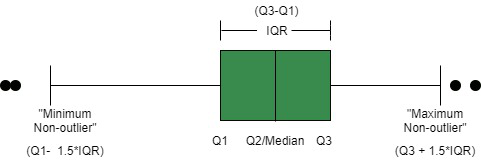In Python 3, We can graph a boxplot using three methods, using matplotlib, using pandas, or using seaborn. Here, we will use seaborn, which is a matplotlib wrapper that provides close integration with pandas data structures and better palette options than matplotlib. We will use seaborn.boxplot() method, and then we will learn how to show mean on boxplot.

Step 1: Importing the libraries and loading the dataset

## Python3

 `# importing useful libraries` `import` `seaborn as sns` `import` `matplotlib.pyplot as plt`   `# using titanic dataset from` `# seaborn library` `df ``=` `sns.load_dataset(``"titanic"``)`   `# to see first 5 rows of dataset` `print``(df.head())`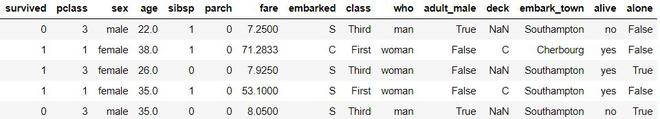First 5 rows of dataset

Step 2: plot a basic boxplot using seaborn.boxplot()

## Python3

 `# to plot a boxplot of` `# age vs survived feature` `plt.figure(figsize``=``(``10``, ``8``))` `sns.boxplot(x``=``'survived'``,` `            ``y``=``'age'``,` `            ``data``=``df)` `plt.ylabel(``"Age"``, size``=``14``)` `plt.xlabel(``"Survived"``, size``=``14``)` `plt.title(``"Titanic Dataset"``, size``=``18``)`

We observe that median is shown as a quartile line, but the mean is not shown.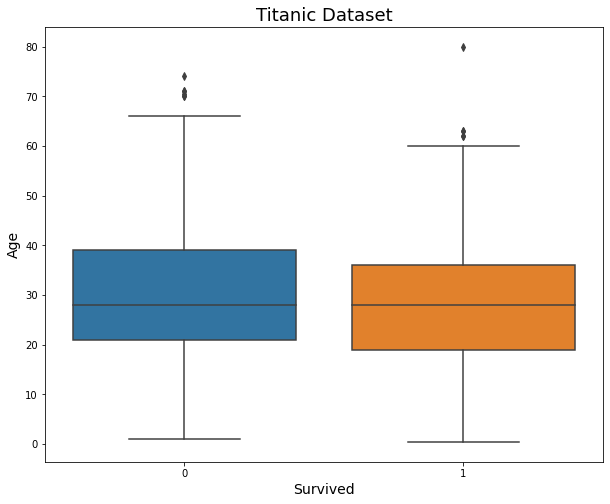simple boxplot

Step 3: To show mean, we use an extra keyword argument in the boxplot function. We set showmeans as True.

## Python3

 `# boxplot with showmeans` `plt.figure(figsize``=``(``10``, ``8``))` `sns.boxplot(x``=``'survived'``,` `            ``y``=``'age'``,` `            ``data``=``df,` `            ``showmeans``=``True``)  ``# notice the change` `plt.ylabel(``"Age"``, size``=``14``)` `plt.xlabel(``"Survived"``, size``=``14``)` `plt.title(``"Titanic Dataset"``, size``=``18``)`

Now, we observe that the mean is marked as a green triangle, which doesn’t go well with our color scheme.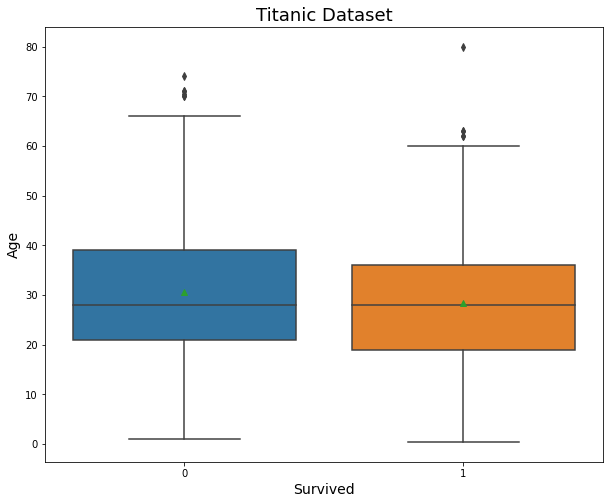plotting mean using showmeans keyword argument

Step 4: To set up our customized marker and markercolor, we will use ‘meanprops’ keyword argument as shown in the code below.

## Python3

 `# customizing using meanprops` `plt.figure(figsize``=``(``10``, ``8``))` `sns.boxplot(x``=``'survived'``,` `            ``y``=``'age'``,` `            ``data``=``df,` `            ``showmeans``=``True``,` `            ``meanprops``=``{``"marker"``: ``"+"``,` `                       ``"markeredgecolor"``: ``"black"``,` `                       ``"markersize"``: ``"10"``})` `plt.ylabel(``"Age"``, size``=``14``)` `plt.xlabel(``"Survived"``, size``=``14``)` `plt.title(``"Titanic Dataset"``, size``=``18``)`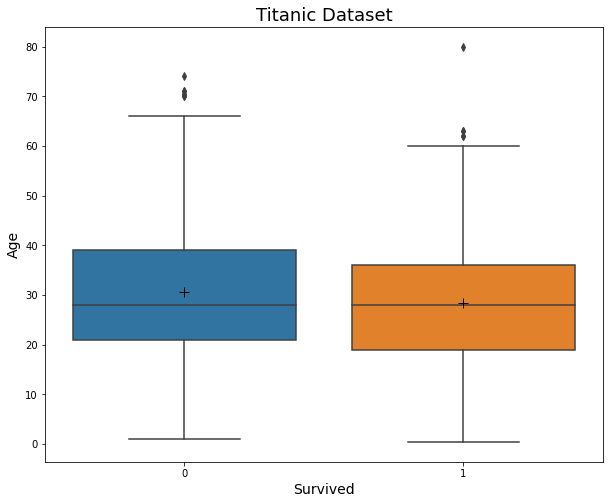My Personal Notes arrow_drop_up
Recommended Articles
Page :